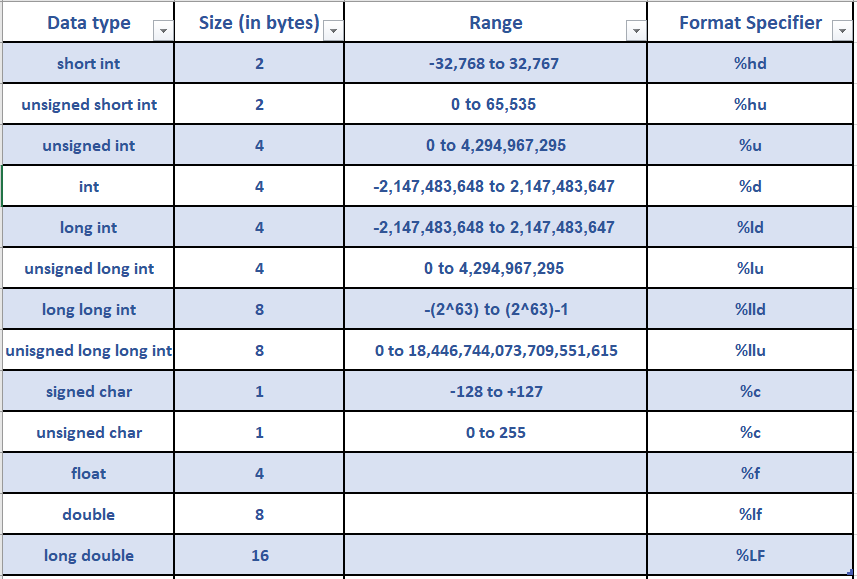In this tutorial, we are going to learn about C data types & data type modifiers.

## Data types in C:

When it comes to computer programming, the data of different formats is represented in binary form (in 0‘s and 1‘s), i.e., in groups of Bits known as Bytes.

For easier and efficient processing, data is classified into different types (data types) such as char, int, float, double, array, enum, etc. These data types can be grouped as primitive and derived.

Primitives form the primary building blocks (they usually have built-in support in the programming languages) and derived data types are usually made up of one or more primitive types. The memory occupied by a variable depends on its data type.

Each data type determines:

• meaning of the data that it represents.
• possible values that it can hold.
• possible operations that can be performed in it.
• the format in which the values of this type are stored.

In C, we need to declare the type of a variable before using it. For example, if we want to store a value 75 in a variable with the name num1. We cannot directly write,

``num1 = 35;``

We first have to declare the variable num1 to be of int type, as mentioned below:

``int num1;``

and then we can assign the values as follows:

``````num1 = 35;
int num1;
num1 = 35;``````

We can also declare and initialize the variable in a single step, as:

``int num1 = 35;``

## Classification of data types in C:## Data type Modifiers:

There are certain keywords in C which, when used along with a given data type can change certain attributes, like memory space occupied by the data type, etc.

For example, storage space for an int data type is 4 bytes in a 32-bit processor. We can increase the storage space to 8 bytes by declaring it as long int. We can decrease space occupied by using short int which will restrict it to 2 bytes.

The below table describes various data types and their size, with their format specifiers:In the above example, long and short keywords are used to change the space allocated to an int data type. As a result, the maximum value that can be stored also changes. The four keywords given below can be used to modify certain attributes of numeric data types:

1. unsigned: This keyword when used, informs that the specified data type can be used to accept only positive values.
2. signed: This keyword when used, informs that the specified data type can accept both negative and positive values. When the keywords unsigned or signed are not used, the system will by default assume the numeric data type to be signed.
3. short: This keyword is used to decrease the size (storage space allocated) to a numeric data type.
4. long: This keyword is used to increase the size (storage space allocated) to a numeric data type.
Note:The term “unsigned” in computer programming indicates a variable that can hold only positive numbers. The term “signed” in computer code indicates that a variable can hold negative and positive values. The property can be applied to most of the numeric data types including int, char, short, and long.

ANSI C has the following rules:

``````short int  <=  int  <=  long int

float   <= double   <= long double``````

These rules show that a short int should be assigned lesser than or the same amount of storage as an int and so on.

## References:

Happy Learning

### About the Author: Hemanth Krishna Vineel RamayanamI’m an Undergraduate Student at the Indian Institute of Information Technology, Design, and Manufacturing, Kurnool, Andhra Pradesh. I belong to the CSE domain.

## One Comment

1.SRINIVAS R January 5, 2021 at 9:06 pm - Reply

Good one…😄👌